# Make Enriched Heatmaps

Author: Zuguang Gu ( z.gu@dkfz.de )

Date: 2017-04-24

Enriched heatmap is a special type of heatmap which visualizes the enrichment of genomic signals to specific target regions. It is broadly used to visualize e.g. how histone modifications are enriched to transcription start sites.

There are several tools that can make such heatmap (e.g. ngs.plot, deepTools or ChIPseeker). Here we implement Enriched heatmap by ComplexHeatmap package. Since this type of heatmap is just a normal heatmap but with some special settings, with the functionality of ComplexHeatmap, it would be much easier to customize the heatmap as well as concatenating to a list of heatmaps to show correspondance between different data sources.

## Basic

``````library(EnrichedHeatmap)
``````

Load the example data that we will use for demostration:

``````set.seed(123)
ls()
``````
``````##  "H3K4me3" "cgi"     "genes"   "meth"    "rpkm"
``````

The example data are all `GRanges` objects:

• `H3K4me3`: coverage for H3K4me3 histone marks
• `cgi`: CpG islands
• `genes`: genes
• `meth`: methylation
• `rpkm`: gene expression

In order to build the vignette fast, the data only includes chromosome 21. Also we downsampled 100000 CpG sites for methylation data.

We first visualize how H3K4me3 histone modification is enriched around TSS. First we extract TSS of genes (note `tss` has strand information):

``````tss = promoters(genes, upstream = 0, downstream = 1)
tss[1:5]
``````
``````## GRanges object with 5 ranges and 0 metadata columns:
##                      seqnames               ranges strand
##                         <Rle>            <IRanges>  <Rle>
##    ENSG00000141956.9    chr21 [43299591, 43299591]      -
##   ENSG00000141959.12    chr21 [45719934, 45719934]      +
##    ENSG00000142149.4    chr21 [33245628, 33245628]      +
##   ENSG00000142156.10    chr21 [47401651, 47401651]      +
##    ENSG00000142166.8    chr21 [34696734, 34696734]      +
##   -------
##   seqinfo: 1 sequence from an unspecified genome; no seqlengths
``````
``````H3K4me3[1:5]
``````
``````## GRanges object with 5 ranges and 1 metadata column:
##       seqnames             ranges strand |  coverage
##          <Rle>          <IRanges>  <Rle> | <integer>
##       chr21 [9825468, 9825470]      * |        10
##       chr21 [9825471, 9825488]      * |        13
##       chr21 [9825489, 9825489]      * |        12
##       chr21 [9825490, 9825490]      * |        13
##       chr21 [9825491, 9825493]      * |        14
##   -------
##   seqinfo: 1 sequence from an unspecified genome; no seqlengths
``````

Similar as other tools, the task of visualization are separated into two steps:

1. get association between genomic signals and targets by normalizing to a matrix.
2. visualize the matrix by heatmap.
``````mat1 = normalizeToMatrix(H3K4me3, tss, value_column = "coverage",
extend = 5000, mean_mode = "w0", w = 50)
mat1
``````
``````## Normalize H3K4me3 to tss:
##   Upstream 5000 bp (100 windows)
##   Downstream 5000 bp (100 windows)
##   Not include target regions
##   720 signal regions
``````
``````class(mat1)
``````
``````##  "normalizedMatrix" "matrix"
``````

`normalizeToMatrix()` converts the association between genomic signals (`H3K4me3`) and targets(`tss`) in to a matrix (actually `mat1` is just a normal matrix with several additional attributes). It first splits the extended targets regions (the extension to upstream and downstream is controlled by `extend` argument) into a list of small windows (the width of the windows is controlled by `w`), then overlaps genomic signals to these small windows and calculates the value for every small window which is the mean value of genomic signals that intersects with the window (the value corresponds to genomic signals are controlled by `value_column` and how to calcualte the mean value is controlled by `mean_mode`).

There are several modes for `mean_mode` according to different types of genomic signals. It will be explained in later sections.

With `mat1`, we can visualize it as a heatmap:

``````EnrichedHeatmap(mat1, name = "H3K4me3")
``````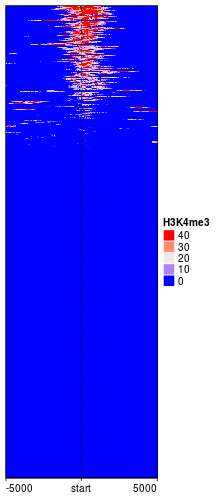`EnrichedHeatmap()` returns an `EnrichedHeatmap` class instance which is inherited from `Heatmap` class, so parameters and methods for `Heatmap` class can be directly applied to `EnrichedHeatmap` class. Users can go to the ComplexHeatmap package to get a more comprehensive help.

### Colors

Similar as the normal heatmap, colors can be controlled by a vector.

``````EnrichedHeatmap(mat1, col = c("white", "red"), name = "H3K4me3")
``````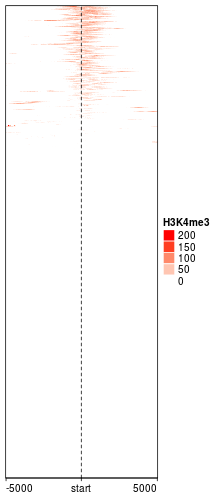You may wonder why the color looks so light. The reason is in coverage values in `H3K4me3`, there exist some extreme values, which results in extreme value in `mat1`.

``````quantile(H3K4me3\$coverage, c(0, 0.25, 0.5, 0.75, 0.99, 1))
``````
``````##   0%  25%  50%  75%  99% 100%
##   10   18   29   43   87  293
``````
``````quantile(mat1, c(0, 0.25, 0.5, 0.75, 0.99, 1))
``````
``````##        0%       25%       50%       75%       99%      100%
##   0.00000   0.00000   0.00000   0.00000  38.96001 166.82353
``````

If a vector of colors is specified, sequential values from minimal to maximal are mapped to the colors, and other values are linearly interpolated. To get rid of such extreme values, there are two ways. The first is to specify `trim` option which trims extreme values both at lower and upper bounds. (In following, it means only to trim values larger than 99th quantile.)

``````mat1_trim = normalizeToMatrix(H3K4me3, tss, value_column = "coverage",
extend = 5000, mean_mode = "w0", w = 50, trim = c(0, 0.01))
EnrichedHeatmap(mat1_trim, col = c("white", "red"), name = "H3K4me3")
``````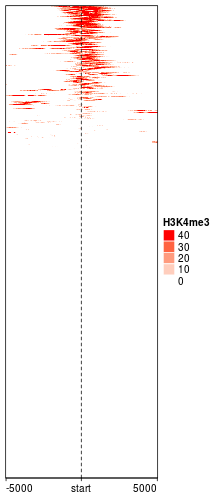The second way is to define a color mapping function which is robust to extreme values. Another advantage of using a color mapping function is that if you have more than one heatmaps to make, it makes colors in heatmaps comparable.

``````library(circlize)
col_fun = colorRamp2(quantile(mat1, c(0, 0.99)), c("white", "red"))
EnrichedHeatmap(mat1, col = col_fun, name = "H3K4me3")
``````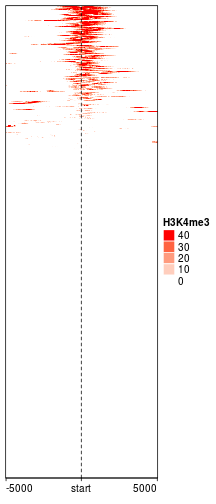To sum it up, the first way directly modified values in `mat1` while the second way keeps the original values but using modified color mappings.

If `col` is not specified in `EnrichedHeatmap()`, blue-white-red is mapped to 1st quantile, median and 99th quantile by default.

In following sections, we will also use the matrix to do row-clustering, thus we directly use the trimmed matrix.

``````mat1 = mat1_trim
``````

### Split on rows

Split rows by a vector or a data frame by specifying `split` option.

``````EnrichedHeatmap(mat1, col = col_fun, name = "H3K4me3",
split = sample(c("A", "B"), length(genes), replace = TRUE),
column_title = "Enrichment of H3K4me3")
``````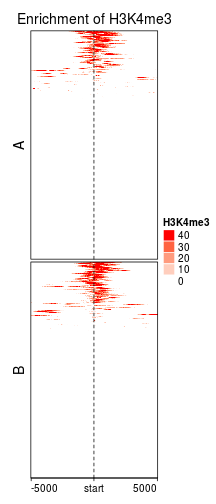Split rows by k-means clustering by specifying `km` option.

``````set.seed(123)
EnrichedHeatmap(mat1, col = col_fun, name = "H3K4me3", km = 3,
column_title = "Enrichment of H3K4me3", row_title_rot = 0)
``````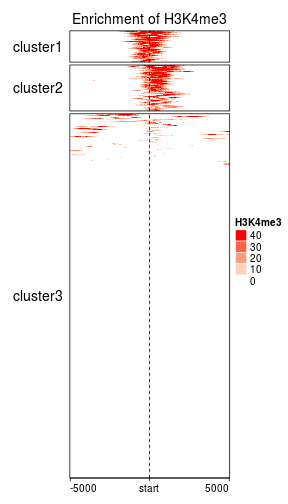### Cluster on rows

Cluster on rows. By default `show_row_dend` is turned off, so you don't need to specify it here. More options for row clustering can be found in the help page of `Heatmap()`.

``````EnrichedHeatmap(mat1, col = col_fun, name = "H3K4me3",
cluster_rows = TRUE, column_title = "Enrichment of H3K4me3")
``````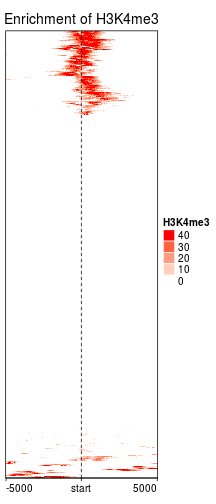### Column annotation

There is a special column annotation function `anno_enriched()` which shows mean values of columns (i.e. mean signals across target regions) in the normalized matrix.

``````EnrichedHeatmap(mat1, col = col_fun, name = "H3K4me3",
top_annotation = HeatmapAnnotation(lines = anno_enriched()),
top_annotation_height = unit(2, "cm"))
``````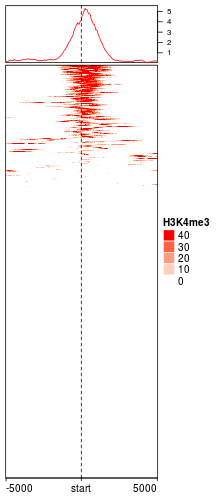If rows are split, the column annotation will show enrichment lines for all row clusters.

``````set.seed(123)
EnrichedHeatmap(mat1, col = col_fun, name = "H3K4me3",
# note we have three row-clusters, so we assign three colors for the annotation lines
top_annotation = HeatmapAnnotation(lines = anno_enriched(gp = gpar(col = 2:4))),
top_annotation_height = unit(2, "cm"),
km = 3, row_title_rot = 0)
``````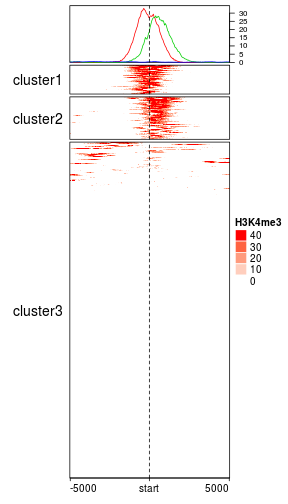You can also add error areas (1 se) for each line. The color of error areas always have the same color of corresponding line but with 75 percent transparency.

Users should be careful with `show_error`. It only makes sense when patterns in heatmap or row clusters are homogeneous.

Actually I am not quite sure whether we should visualize the errors because the spreadness of the data can already be seen in the heatmaps.

Also we demonstrate how to add legend for the annotation lines by `Legend()` function from ComplexHeatmap package.

``````set.seed(123)
lgd = Legend(at = c("cluster1", "cluster2", "cluster3"), title = "Clusters",
type = "lines", legend_gp = gpar(col = 2:4))
ht = EnrichedHeatmap(mat1, col = col_fun, name = "H3K4me3",
# note we have three row-clusters, so we assign three colors for the annotation lines
top_annotation = HeatmapAnnotation(lines = anno_enriched(gp = gpar(col = 2:4), show_error = TRUE)),
top_annotation_height = unit(2, "cm"),
km = 3, row_title_rot = 0)
draw(ht, annotation_legend_list = list(lgd))
``````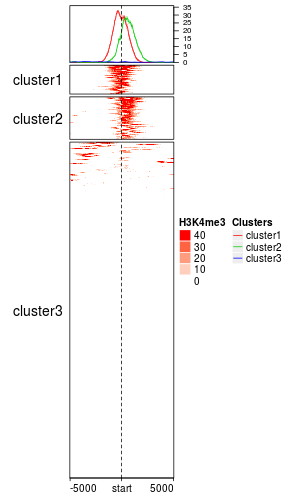### Smoothing

Rows can be smoothed by setting `smooth` to `TRUE` when generating the matrix. Later we will demonstrate smoothing can also help to impute `NA` values.

As smoothing may change the original data range, the color mapping function `col_fun` here ensures that the color palette is still the same as the unsmoothed one.

`empty_value` corresponds to the regions that have no signal overlapped. The proper value depends on specific scenarios. Here since we visualize coverage from ChIP-Seq data, it is reasonable to assign 0 to regions with no H3K4me3 signal.

``````mat1_smoothed = normalizeToMatrix(H3K4me3, tss, value_column = "coverage",
extend = 5000, mean_mode = "w0", w = 50, empty_value = 0, smooth = TRUE)
EnrichedHeatmap(mat1_smoothed, col = col_fun, name = "H3K4me3")
``````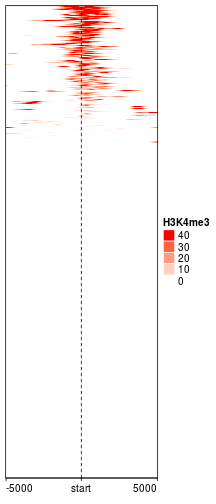### Extensions to target regions

Extension to upstream and downstream can be controled by `extend` either by a single value or a vector of length 2.

``````# upstream 1kb, downstream 2kb
mat12 = normalizeToMatrix(H3K4me3, tss, value_column = "coverage",
extend = c(1000, 2000), mean_mode = "w0", w = 50)
EnrichedHeatmap(mat12, name = "H3K4me3", col = col_fun)
``````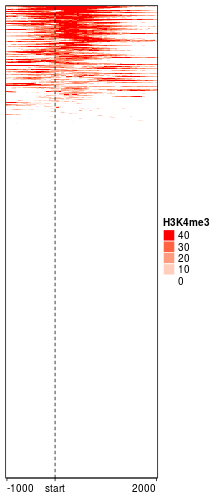Either upstream or downstream can be set to 0.

``````mat12 = normalizeToMatrix(H3K4me3, tss, value_column = "coverage",
extend = c(0, 2000), mean_mode = "w0", w = 50)
EnrichedHeatmap(mat12, name = "H3K4me3", col = col_fun)
``````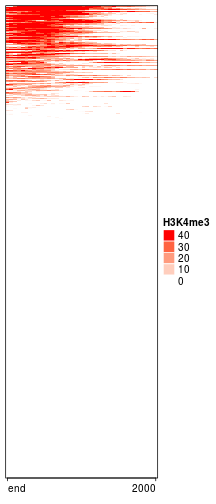``````mat12 = normalizeToMatrix(H3K4me3, tss, value_column = "coverage",
extend = c(1000, 0), mean_mode = "w0", w = 50)
EnrichedHeatmap(mat12, name = "H3K4me3", col = col_fun)
``````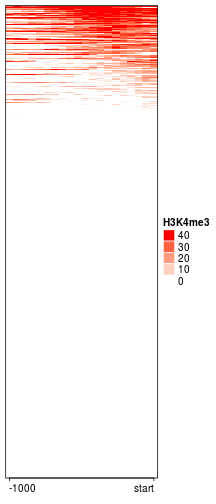### Other graphic settings

By default there is an axis on the bottom border of the heatmap and a vertical line which represents the targets. There are several arguments which can be used to customize:

``````EnrichedHeatmap(mat1, col = col_fun, name = "H3K4me3",
pos_line_gp = gpar(col = "blue", lwd = 2), axis_name = c("-5kb", "TSS", "5kb"),
axis_name_rot = -45, border = FALSE)
``````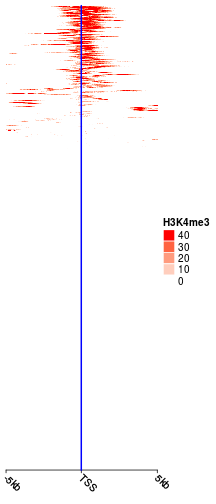### Mean mode

Upstream and downstream also the target body are segmented into a list of small windows and overlap to signal regions. Since signal regions and small windows do not always 100 percent overlap, to summarize values in small windows, there are four different average modes:

Following illustrates different settings for `mean_mode` (note there is a signal region overlapping with other signal regions):

``````   40      50     20     values in signal
++++++   +++    +++++   signal
30               values in signal
++++++             signal
=================     window (17bp), there are 4bp not overlapping to any signal region.
4  6  3      3      overlap

absolute: (40 + 30 + 50 + 20)/4
weighted: (40*4 + 30*6 + 50*3 + 20*3)/(4 + 6 + 3 + 3)
w0:       (40*4 + 30*6 + 50*3 + 20*3)/(4 + 6 + 3 + 3 + 4)
coverage: (40*4 + 30*6 + 50*3 + 20*3)/17
``````

To explain it more clearly, let's consider two scenarios:

First, we want to calculate mean methylation from 3 CpG sites in a 20bp window. Since methylation is only measured at CpG site level, the mean value should only be calculated from the 3 CpG sites while not the non-CpG sites. In this case, `absolute` mode should be used here.

Second, we want to calculate mean coverage in a 20bp window. Let's assume coverage is 5 in 1bp ~ 5bp, 10 in 11bp ~ 15bp and 20 in 16bp ~ 20bp. Since converage is kind of attribute for all bases, all 20 bp should be taken in account. Thus, here `w0` mode should be used which also takes account of the 0 coverage in 6bp ~ 10bp. The mean coverage will be caculated as `(5*5 + 10*5 + 20*5)/(5+5+5+5)`.

Third, genes have multiple transcripts and we want to calculate how many transcripts eixst in a certain position in the gene body. In this case, values associated to each transcript are binary (either 1 or 0) and `coverage` mean mode should be used.

### Smoothing improves visualization on methylation profiles

Following heatmap visualizes the enrichment of low methylated regions on TSS. The grey colors represent the windows with no CpG sites (note we set `NA` to `empty_value` and grey is the default color for `NA` values by ComplexHeatmap).

``````meth[1:5]
``````
``````## GRanges object with 5 ranges and 1 metadata column:
##       seqnames             ranges strand |              meth
##          <Rle>          <IRanges>  <Rle> |         <numeric>
##       chr21 [9432427, 9432427]      * | 0.267104203931852
##       chr21 [9432428, 9432428]      * | 0.267106771955287
##       chr21 [9432964, 9432964]      * | 0.272709911227985
##       chr21 [9432965, 9432965]      * |   0.2727345344132
##       chr21 [9433315, 9433315]      * | 0.285114797969136
##   -------
##   seqinfo: 1 sequence from an unspecified genome; no seqlengths
``````
``````mat2 = normalizeToMatrix(meth, tss, value_column = "meth", mean_mode = "absolute",
extend = 5000, w = 50, empty_value = NA)
meth_col_fun = colorRamp2(c(0, 0.5, 1), c("blue", "white", "red"))
EnrichedHeatmap(mat2, col = meth_col_fun, name = "methylation", column_title = "methylation near TSS")
``````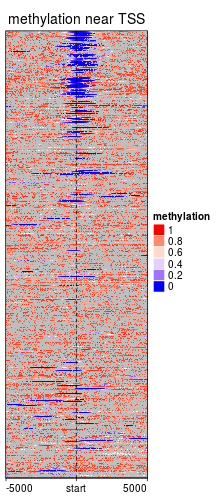When overlapping CpG positions to segmented target regions, it is possible that there is no CpG site in some windows. The values for these windows which contain no CpG sites can be imputed by smoothing. Although it seems not proper to assign methylation values to non-CpG windows, but it will enhance the effect of visualization a lot.

``````mat2 = normalizeToMatrix(meth, tss, value_column = "meth", mean_mode = "absolute",
extend = 5000, w = 50, empty_value = NA, smooth = TRUE)
EnrichedHeatmap(mat2, col = meth_col_fun, name = "methylation", column_title = "methylation near TSS")
``````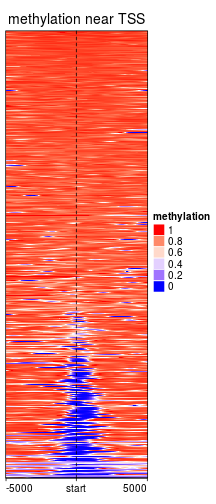To do the smoothing, by default, `locfit()` is first applied to each row in the original matrix. If it is failed, `loess()` smoothing is applied afterwards. If both smoothing methods are failed, there will be a warning and the original value is kept.

Users can provides their own smoothing function by `smooth_fun` argument. This self-defined function accepts a numeric vector (may contains `NA` values) and returns a vector with same length. If the smoothing is failed, the function should call `stop()` to throw errors so that `normalizeToMatrix()` can catch how many rows are failed in smoothing. Take a look at the source code of `default_smooth_fun()` to get an example.

## Targets are regions

In the example of H3K4me3, the target regions are single points. The target can also be regions with width larger than 1. Following heatmap visualizes the enrichment of low methylation on CpG islands:

``````mat3 = normalizeToMatrix(meth, cgi, value_column = "meth", mean_mode = "absolute",
extend = 5000, w = 50, empty_value = NA, smooth = TRUE)
EnrichedHeatmap(mat3, col = meth_col_fun, name = "methylation", column_title = "methylation near CGI")
``````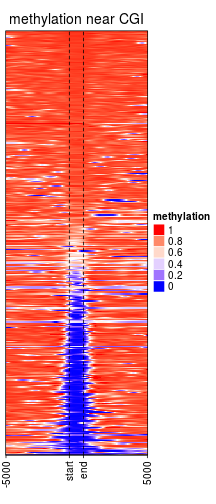Width of the target regions shown on heatmap can be controlled by `target_ratio` which is relative to the width of the complete heatmap.

Target regions are also splitted into small windows, but since width of the target regions are different from each other, they are splitted by percent to their full width (the percent value is calculated automatically).

``````mat3 = normalizeToMatrix(meth, cgi, value_column = "meth", mean_mode = "absolute",
extend = 5000, w = 50, empty_value = NA, smooth = TRUE, target_ratio = 0.3)
EnrichedHeatmap(mat3, col = meth_col_fun, name = "methylation", axis_name_rot = 90,
column_title = "methylation near CGI")
``````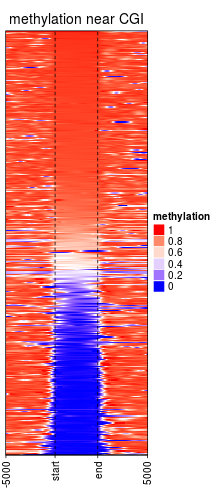When genomic targets are regions, upstream and/or downstream can be excluded in the heatmap.

``````mat3 = normalizeToMatrix(meth, cgi, value_column = "meth", mean_mode = "absolute",
extend = c(0, 5000), w = 50, empty_value = NA, smooth = TRUE, target_ratio = 0.5)
EnrichedHeatmap(mat3, col = meth_col_fun, name = "methylation", axis_name_rot = 90,
column_title = "methylation near CGI")
``````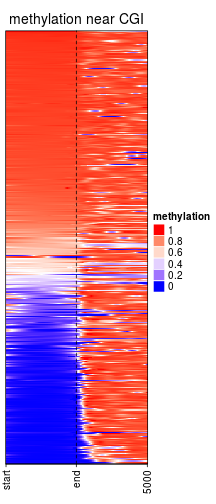``````mat3 = normalizeToMatrix(meth, cgi, value_column = "meth", mean_mode = "absolute",
extend = c(5000, 0), w = 50, empty_value = NA, smooth = TRUE, target_ratio = 0.5)
``````
``````## Warning in normalizeToMatrix(meth, cgi, value_column = "meth", mean_mode = "absolute", :
## Smoothig is failed for one row because there are very few signals overlapped to it.
## Please use `attr(mat, 'failed_rows')` to get the index of the failed row and consider to
## remove it.
``````
``````EnrichedHeatmap(mat3, col = meth_col_fun, name = "methylation", axis_name_rot = 90,
column_title = "methylation near CGI")
``````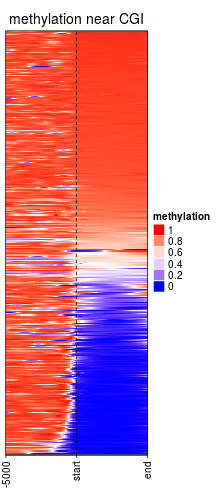``````# since there is not upstream and downstream, the number of columns is controlled by `k` argument
mat3 = normalizeToMatrix(meth, cgi, value_column = "meth", mean_mode = "absolute",
extend = 0, k = 20, empty_value = NA, smooth = TRUE, target_ratio = 1)
``````
``````## Warning in normalizeToMatrix(meth, cgi, value_column = "meth", mean_mode = "absolute", :
## Smoothig is failed for one row because there are very few signals overlapped to it.
## Please use `attr(mat, 'failed_rows')` to get the index of the failed row and consider to
## remove it.
``````
``````EnrichedHeatmap(mat3, col = meth_col_fun, name = "methylation", axis_name_rot = 90,
column_title = "methylation near CGI")
``````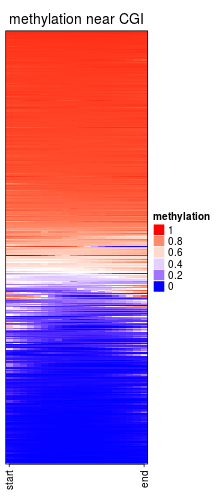You may notice there are warnings when executing above code, that is because there are very few signals overlapped to some rows, which means there are too many `NA` to do the smoothing. Corresponding index for failed rows can be get by :

``````attr(mat3, "failed_rows")
``````
``````##  5
``````

and maybe you can remove them beforehand.

## Multiple heatmaps

The power of EnrichedHeatmap package is that parallel heatmaps can be concatenated, both for enriched heatmap, normal heatmap as well the normal row annotations, which provides a very efficient way to visualize multiple sources of information.

With the functionality of ComplexHeatmap package, heatmaps can be concatenated by `+` operator. `EnrichedHeatmap` objects, `Heatmap` objects and `HeatmapAnnotation` objects can be mixed.

Following heatmaps visualizes correspondance between H3K4me3 modification, methylation and gene expression. It is quite straightforward to see high expression correlates with low methylation and high H3K4me3 signal around TSS.

``````EnrichedHeatmap(mat1, col = col_fun, name = "H3K4me3", width = 1) +
EnrichedHeatmap(mat2, col = meth_col_fun, name = "methylation", width = 1) +
Heatmap(log2(rpkm+1), col = c("white", "orange"), name = "log2(rpkm+1)",
show_row_names = FALSE, width = unit(5, "mm"))
``````